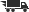Close

# Calculus for Engineers and Scientists, Volume 1

## by Giordano, Frank R.Weir, Maurice D.Finney, Ross L.

• ISBN: 9780201442052
• ISBN10: 0201442051

# Calculus for Engineers and Scientists, Volume 1

## by Giordano, Frank R.Weir, Maurice D.Finney, Ross L.

• List Price: \$67.00
• Binding: Paperback
• Edition: 1
• Publish date: 12/18/1997
• ISBN: 9780201442052
• ISBN10: 0201442051
new \$68.23
Product notice Returnable at the third party seller's discretion and may come without consumable supplements like access codes, CD's, or workbooks.
Description: (Each chapter ends with Practice Exercises and Additional Exercises-Theory, Examples, Applications.) VOLUME ONE 1. Limits and Continuity. Limits and Rates of Change. Rules for Finding Limits. Continuity. Tangent Lines. Writing for Your Review. 2. Derivatives. The Derivative as a Function. The Derivative as a Rate of Change. Products, Quotients, and Negative Powers. Derivatives of Trigonometric Functions. The Chain Rule. Implicit Differentiation and Rational Exponents; Related Rates. Writing for Your Review. 3. Extreme Values and Differential Equations. Extreme Values of Functions. The Mean Value Theorem and Differential Equations. The Shape of a Graph. Graphical Solutions to Differential Equations. Exponential Functions and the Derivative of ex. Writing for Your Review. 4. Initial Value Problems. Indefinite Integrals, Differential Equations, and Modeling. Integral Rules; Integration by Substitution. First Order Differential Equations. Vectors in the Plane. Modeling Projectile Motion. Questions to Guide Your Review. 5. Definite Integrals. Estimating with Finite Sums. Riemann Sums and Definite Integrals. The Fundamental Theorem. The Natural Logarithm. Area. Substitution in Definite Integrals; Properties. The Mean Value Theorem and Proof of the Fundamental Theorem. Numerical Integration. Questions to Guide Your Review. 6. Applications of Derivatives and Integrals. Optimization. Linearization and Differentials. Newton''s Method. Volume. Springs, Pumping, and Lifting. Fluid Forces. Questions to Guide Your Review. 7. Applications of Differential Equations. Growth and Decay; Linear First Order Differential Equations. Euler''s Numerical Method. Models for Springs and Pendulums. Constant-Coefficient Homogeneous Second Order Linear Equations. Unforced Oscillatory Motion. Writing for Your Review. 8. Inverse Trigonometric Functions. Inverse Functions and Their Derivatives. Inverse Trigonometric Functions. Derivatives of Inverse Trigonometric Functions; Integrals. Writing for Your Review. 9. Techniques of Integration. Basic Integration Formulas. Integration by Parts-Running the Product Rule Backwards. Partial Fractions. Integration with a Computer Algebra System (CAS). Numerical Integration: The Monte Carlo Method. Questions to Guide Your Review. (Each chapter ends with Writing for Your Review, Practice Exercises, and Additional Exercises-Theory, Examples, Applications.) VOLUME TWO 10. Vectors in Space. Vectors in Space. Dot Products. Cross Products. Lines and Planes in Space. Space Curves. Arc Length and the Unit Tangent Vector T. The TNB Frame; Tangential and Normal Components of Acceleration. 11. Partial Derivatives. Functions, Limits, and Continuity. Partial Derivatives. The Chain Rule. Directional Derivatives, Gradient Vectors, and Tangent Planes. Linearization and Differentials. Partial Derivatives with Constrained Variables. 12. Multiple Integrals. Double Integrals. Areas, Moments, and Center of Mass. Double Integrals in Polar Form. Triple Integrals in Rectangular Coordinates. Masses and Moments in Three Dimensions. Triple Integrals in Cylindrical and Spherical Coordinates. Substitutions in Multiple Integrals. 13. Integration in Vector Fields. Line Integrals. Vector Fields, Work, Circulation, and Flux. Path Independence, Potential Functions, and Conservative Fields. Green''s Theorem in the Plane. Surface Area and Surface Integrals. Parametrized Surfaces. Stokes''s Theorem. The Divergence Theorem and a Unifed Theory. 14. Extreme Values and Extensions of the Limit Concept. Extreme Values and Saddle Points for Multivariable Functions. Lagrange Multipliers. Extensions of the Limit Concept. L''Hpital''s Rule. Improper Integrals. 15. Power Series. Power Series Representations of Functions. Taylor Series. Taylor''s Theorem. Radius of Convergence. Convergence at Endpoints.
Expand description
Product notice Returnable at the third party seller's discretion and may come without consumable supplements like access codes, CD's, or workbooks.
 Seller: Media Smart ★★★★★ ★★★★★ Location: Hawthorne, CA Condition: NewP 372. P 372. Price: \$68.23 Comments: P 372. P 372.